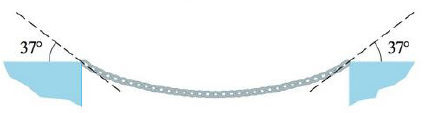Chapter 12.2, Problem 38E

Chapter
Section
Textbook Problem

# The tension T at each end of a chain has magnitude 25 N (see the figure). What is the weight of the chain?To determine

To find: The weight of the chain.

Explanation

Given:

The magnitude of chain at each end as 25 N and angle at each end is 37° .

Formula used:

Write the expression for tension vector in terms of horizontal and vertical components (T) .

T=|T|cosθi+|T|sinθj (1)

Here,

|T| is magnitude of tension, and

θ is angle of tension.

The weight of chain is counterbalanced by the combined tension contributed by tension at ends of chain.

Write the expression for weight of chain (w) .

|w|j=T1+T2 (2)

Here,

|w| is magnitude of weight,

T1 is tension vector of first end of chain, and

T2 is tension vector of second end of chain.

Draw the vector diagram of chain as shown in Figure 1.

Find the tension vector at first end of chain by using equation (1).

T1=(25N)cos(37°)i+(25N)sin(37°)j=(25)(0.7896)i+(25)(0.6018)j=19.740i+15.045j

Find the tension vector at second end of chain by using equation (1)

### Still sussing out bartleby?

Check out a sample textbook solution.

See a sample solution

#### The Solution to Your Study Problems

Bartleby provides explanations to thousands of textbook problems written by our experts, many with advanced degrees!

Get Started

#### Find more solutions based on key concepts